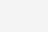# 如何在Core i5 上实现 20 倍的 Python 运行速度？James Reinders

James Reinders：利用 Intel Distribution for Python，我实现了 Python 的 20 倍加速，并且可用单个命令关闭/启用。这还不是在最优情况下，而在虚拟环境——在 VirtualBox（下文简称 VBox） 上运行的 openSUSE Linux Tumbleweed（即“滚动版本”，请参考 openSUSE 官网)，使用的机器是四核 iMac。

% source deactivate intelpy

% Python < myprog.py

np.sin

102400 10000 36.1987440586

np.cos

102400 10000 36.1938228607

np.tan

102400 10000 51.487637043

% source activate intelpy

% Python < myprog.py

np.sin

102400 10000 1.76131296158

np.cos

102400 10000 1.83870100975

np.tan

102400 10000 3.38778400421

That s all!  The speed-ups are 20.6X, 19.7X, and 15.2X in this quick test running on a virtual machine.

Here s my little Python program:

% cat myprog.py

import numpy as np

import time

N = 102400

x = np.linspace(0.0123, 4567.89, N)

def mine(x,Z,func,name):

print name;

start = time.time()

for z in range ( 0, Z ) :

y = func(x);

end = time.time()

print N, Z, end - start

return

mine(x,10000,np.sin,'np.sin')

mine(x,10000,np.cos,'np.cos')

mine(x,10000,np.tan,'np.tan')

Intel Distribution for Python 带来的大幅加速能力，让 “accelerated Python” 变得更实用更让人信服。

FFT 在 4 核虚拟机上有八倍性能提升

% source deactivate intelpy

% Python < myfftprog.py

fft

5000 2.22796392441

fft

7000 8.74916005135

% source activate intelpy

% Python < myfftprog.py

fft

5000 0.277629137039

fft

7000 1.11230897903

% cat myfftprog.py

import numpy as np

import numpy.random as rn

import time

def trythis(Z):

mat = rn.rand(Z,Z) + 1j * rn.randn(Z,Z)

print 'fft'

start = time.time()

# 2D transform on a complex-valued matrix:

result = np.fft.fft2(mat)

end = time.time()

print Z, end - start

return

trythis(5000);

trythis(7000);

Transcendental expressions 包含我在快速示例程序里用的 cosine, sine 和 tangen。这些优化的核心是对 NumPy 的改变，使得 primitives （在 ndarray 数据上进行运算）能选择性地使用英特尔 MKL Short Vector Math Library (SVML) 和 MKL Vector Math Library (VML) 的能力。这使得 Python 利用处理器的最新矢量能力，包括多核优化和 AVX/AVX2/AVX-512。英特尔团队表示，他们利用 Xeon Phi，实现过 NumPy 算术和 transcendental 运算在 vector-vector 和 vector-scalar 上最高 400 倍的速度提升。

Python 是一门动态语言，为用户管理内存。Python 应用的性能，在很大程度上取决于内存运行的性能，这包括内存分配、再分配（de-allocation）、复制和移动。英特尔提供的加速版本 Python，能在 NumPy 分配数组时保证最佳的  alignment，所以 NumPy、SciPy 的运算函数，能从相应排列的 SIMD 内存访问指令获益。英特尔表示最大的提升来自于对内存复制和移动运算的优化。

Anaconda 英特尔渠道的最新加速版本 Python，为 Python 程序带来显著性能优化，而无需改变代码。下载、安装也很方便。

via infoworld